# The Modern Periodic Table Notes | Study Science Class 10 - Class 10

## Class 10: The Modern Periodic Table Notes | Study Science Class 10 - Class 10

The document The Modern Periodic Table Notes | Study Science Class 10 - Class 10 is a part of the Class 10 Course Science Class 10.
All you need of Class 10 at this link: Class 10

In 1913 Henry Moseley showed that properties of the elements are determined by atomic numbers instead of the atomic mass. It formed the basis of modern periodic law. The law is -

"The physical and chemical properties of the elements are periodic function of their atomic numbers".
Since atomic mass is a nuclear property whereas atomic number implies for the no. of electrons in neutral atom or no. of protons in nucleus. Nucleus is deep seated in the atoms and does not take part in chemical reactions. Therefore the physical and chemical properties depends upon the no. of electrons and their electronic configuration which in turn depends upon atomic number (Z). So when elements are arranged in the increasing order of atomic numbers, after a regular interval elements have similar no. of valence electrons therefore chemical properties are repeated i.e. periodicity in the chemical properties of the elements occurs.

Modern Periodic Table

It is also called as Bohr, Bury & Rang, Werner periodic table.

(1) It is based on the Bohr-Bury electronic configuration concept and atomic number.

(2) This model is proposed by Rang and Werner.

This table is based on modern periodic law, the elements are arranged in the increasing order of atomic numbers in such a way that elements having the same number of valence electrons are placed in the same vertical column.

It consists of 18 vertical columns and seven horizontal rows. Vertical columns of periodic table are known as groups while horizontal rows are known as periods.

The co-relation between the groups in long form of periodic table and in modern form of periodic table are given below:

 IA IIA IIA IVB VB VIB VIIB VII IB IIB 1 2 3 4 5 6 7 8.9. 10 11 12 IIIA IV A VA VIA VIIA 0 13 14 15 16 17 18

Elements belonging to same group have same number of electrons in the outer most shell so their properties are similar.

Description of periods :

 Period n Sub shell No. of elements Element Name of Period 1. 1 1s 2 1H, 2He Shortest 2. 2 2s,2p 8 3Li – 10Ne Short 3. 3 3s, 3p 8 11Na – 18Ar Short 4. 4 4s, 3d, 4p 18 19K – 36Kr Long 5. 5 5s, 4d, 5p 18 37Rb – 58Xe Long 6. 6 6s, 4f, 5d, 6p 32 55Cs – 86Rn Longest 7. 7 7s, 5f, 6d, 26 87Fr – 112Uub Incomplete

Description of Groups

1st/IA/Alkali metals
H = 1s1
Li = 1s2 , 2s1
Na = 1s2 , 2s2 2p6 , 3s1
K = 1s2 , 2s2 2p6 , 3s2 3p6 , 4s1

General electronic configuration = ns(n = Number of shell)

Number of valence shell e = 1

2nd/IIA/Alkali earth metals :

Be = 1s2, 2s2
Mg = 1s2, 2s2, 2p6, 3s2
Ca = 1s2, 2s2, 2p6, 3s2, 3p6, 4s2

General electronic configuration = ns2

Number of valence shell es = 2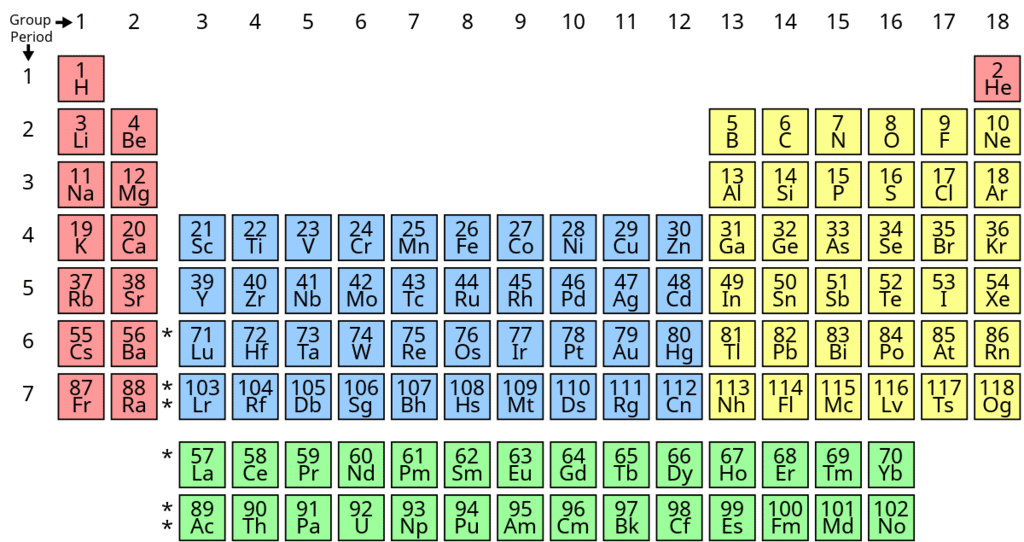13th/IIIA/Boron Family :
B = 1s2, 2s2, 2p1
Al = 1s2, 2s2, 2p6, 3s2, 3p1
Ga = 1s2, 2s2, 2p6, 3s2, 3p6, 3d10,4s2,4p1

General electronic configuration = ns2 np1

Number of valence shell e s = 3

14th/IVA/Carbon Family :

C = 1s2, 2s2, 2p2
Si = 1s2, 2s2, 2p6, 3s2, 3p2
Ge = 1s2, 2s2, 2p6, 3s2, 3p6, 4s2, 3d10, 4p2
General electronic configuration = ns2 np2

Number of valence e s= 4

15th/VA/Nitrogen family/Pnicogen : (Used in fertilizer as urea)

N = 1s2, 2s2, 2p3
P = 1s2, 2s2, 2p6, 3s2, 3p3
As = 1s2, 2s2, 2p6, 3s2, 3p6, 4s2, 3d10, 4p3

General electronic configuration = ns2 np3

Number of valence shell e = 5

16th/VIA/Oxygen family/Chalcogen : (Ore forming)

O = 1s2, 2s2, 2p4

S = 1s2, 2s2, 2p6, 3s2, 3p4

Se = 1s2, 2s2, 2p6, 3s2, 3p6,3d10, 4s2, 4p4

General electronic configuration : ns2 np4

Number of valence shell e s= 6

17th/VIIA/Fluorine family/Halogens : (Salt forming)

F = 1s2, 2s2, 2p5

Cl = 1s2, 2s2, 2p6, 3s2, 3p5

Br = 1s2, 2s2, 2p6, 3s2, 3p6, 4s2, 3d10, 4p5

General electronic configuration = ns2 np5

Number of valence shell e s= 7

18th/Zero group/Inert gases / Noble gases :

Ne = 1s2, 2s2, 2p6

Ar = 1s2, 2s2, 2p6, 3s2, 3p6

Kr = 1s2, 2s2, 3p6, 3s2, 3p6, 3d10,4s2, 4p6

General electronic configuration = ns2 np6 ( except. He)

Number of valence shell e = 8

Elements of group 16 are known as chalcogens. Elements of group 17 are known as halogens.

Trends in Modern Periodic Table

The electronic configurations of atoms display a periodic variation with increase in atomic number. Since the properties of elements depends upon the electronic configurations. So the elements exhibits periodic variation of physical & chemical properties. Some properties of elements are:

Valency

It is defined as the combining capacity of the element. Valency is determined by the number of electrons present in outer most shell. These electrons are known as valence electrons.

Variation of valency across a period :- The number of valence electrons increases from 1 to 8 on moving across a period. The valency of an element with respect to hydrogen and halogen increases from 1 to 4 and then decreases from 4 to zero. With respect to oxygen valency increases from 1 to 7.

Variation of valency along a group :- On moving down a group. The no. of valence electrons remains same. So the valency of all the elements of a group is same.

Group (1) elements have valency - 1

Group (2) elements have valence - 2

Atomic size

Atomic size means atomic radius of an atom which is defined as the distance between the centre of the nucleus of an atom and the valence shell containing electrons in an isolated atom, since it is very difficult to measure the atomic radius because -

(i) The isolation of single atom is very difficult.
(ii) There is no well-defined boundary for the atom.

So, the more accurate definition of atomic radius is -

(Half the internuclear distance between the two atoms in a homoatomic molecule is known as atomic radius). This internuclear distance is also known as bond length. It depends upon the type of bond by which two atoms combine.

Based on chemical bonds, atomic radius is divided in to four categories:

(a) Covalent radius (Single bonded covalent radius): For homoatoms, it is half of the internuclear distance between two singly bonded homoatoms.

(b) Covalent radius for hetero atoms:

(i) In case of hetero atomic molecule (A – B), if the electronegativity difference is less. Then covalent radius of oxygen, nitrogen and carbon is taken from the compound H2O2, N2H4 and C2H6 respectively.

This radii is subtracted from the bond length of A–B molecules.
eg. C–I (electronegativity is almost same 2.5)

Internuclear distance C–I is 2.13Å, covalent radius of carbon in compound C2H6 is 0.77Å covalent radius of I– will be:
dC–I = rC (covalent radius of carbon) + rI (covalent radius of iodine)
i.e. 2.13 = 0.77 + rI

rI = 2.13 – 0.77 = 1.36Å

(ii) When electronegativity difference is more. Then bond length is determined by the schole maker and Stevenson law –
dA–B = rA + rB – 0.09(XA – XB)
where dA–B = Bond length of dA–B molecule
XA = Electronegativity of A
XB = Electronegativity of B

Example – Bond length of F2 = 1.44 Å
i,e. dF–F = 1.44Å

rF = (1.44/2)= 0.72Å

dH–H = 0.74Å

rH =(0.74/2)= 0.37Å

Electronegativity of Fluorine is 4.0 and Electronegativity of Hydrogen is 2.1.

dH–F = rF + rH + 0.09(XF – XH)

= 0.72 + 0.37 – 0.09(4 – 2.1)

= 1.09 – (0.09 × 1.9)

= 1.09 – 0.171 = 0.919Å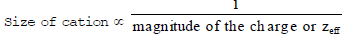eq. Fe > Fe+2 > Fe+3

Anionic radius is always greater than atomic radius because in an anion electrons are more than the protons so effective nuclear charge reduces and inter electronic repulsion increases so size of anion also increases.

Half of the inter nuclear distance between two adjacent metallic atoms.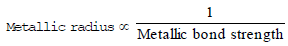Those atoms which are not bonded with each other experience a weak attractive force to come nearer. Half of the distance between the nuclei of adjacently placed atoms in solid state of a noble gas is Vander waal's radius.

Variation of Atomic size in a group:

On moving down a group of periodic table, the size of the atom increases.

Variation of atomic size in period: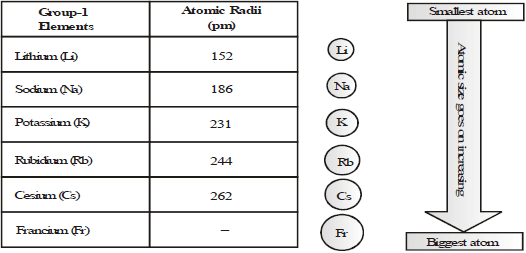When we move from top to bottom in a group, a new shell of electron is added in each period. This addition increases the size.

In general atomic radii decreases across a period from left to right eg. In IInd period.

Li atom is largest and Fluorine is the smallest atom because nuclear charge increases with increase in atomic number. Electrons are also increasing but these are added to the same shell.

 Element Li Be B C N O F Atomic Number 3 4 5 6 7 8 9 Nuclear charge + 3 +4 + 5 + 6 + 7 + 8 + 9 Electronic configuration 2, 1 2, 1 2, 3 2, 4 2, 5 2, 6 2, 7 Radius (pm) 152 111 88 77 74 66 64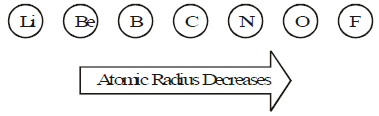Atomic Size :- Decreases along the period. Increases down the group.

Metallic and Non-metallic Character

Metallic character is the tendency of atoms of the elements to lose electrons and form positive ions. It can be expressed as
M → M+ + e

Therefore metals are also called as electropositive elements.
The metallic character increases from top to bottom in a group. Eg. Li is least metallic element while Caesium is most metallic element.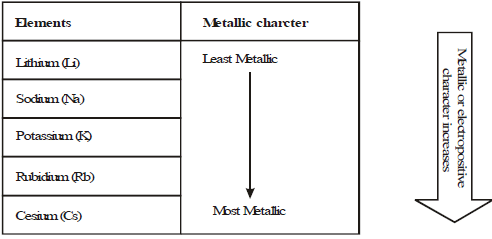If we use the term electropositive in place of metallic character, we can say that electropositive character goes on increasing as we move from top to bottom in the periodic table. If we consider the electronegative character, it goes on decreasing as we move down in a group of the periodic table.

Ionization Enthalpy:

The minimum amount of energy required to remove the most losely bounded electron from an isolated gaseous neutral atom to form gaseous electropositive ion is called Ionization enthalpy. Its unit is kilo joules per mole (kJ/mol)
M(g) + Energy → M+
It is a measure of tendency of atoms to lose electrons. The tendency to lose electron increases from top to bottom in a group and it decreases on moving left to right in a period.

Electron gain enthalpy:

It is defined as the amount of energy released when an isolated gaseous atom in the ground state accepts an electron to form gaseous negative ion i.e. an anion. It is a measure of tendency of an atom to accept an extra electron to form an anion. Its unit is kilo joule per mole (kJ/mole). Electron gain enthalpy of elements goes on increasing as we move from left to right in a period. In group it decreases  from top to bottom.

The document The Modern Periodic Table Notes | Study Science Class 10 - Class 10 is a part of the Class 10 Course Science Class 10.
All you need of Class 10 at this link: Class 10Use Code STAYHOME200 and get INR 200 additional OFF

## Science Class 10

107 videos|325 docs|162 tests

Track your progress, build streaks, highlight & save important lessons and more!

,

,

,

,

,

,

,

,

,

,

,

,

,

,

,

,

,

,

,

,

,

;